Examples for AC circuits

Example #1

Problem:

An AC circuit carries an rms current of 7.0 Amps. The current travels through a 12 Ohm resistor.

a.) What is the peak current?

Solution: Multiply the rms current by sqrt(2).

I = 9.90 A

b.) What is the power dissipated in the resistor?

Solution: You can use the normal formula, i2R, provided that you use the rms value of the current.

588 W

c.) What is the peak voltage drop across the resistor?

Solution: Use Ohm's law, being careful to use the peak current to get the peak voltage.

118.8 V

Example #2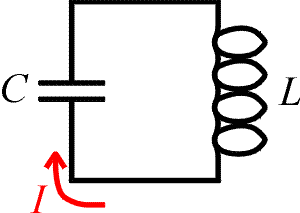Problem:

Consider the LC circuit to the right. If one needs to tune this circuit to a frequency of 84 kHz, and the capacitor has a capacitance C = 3.0 mF, what inductance L is needed?

Solution:

Use the relation,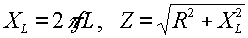. Solve for L.

L = 1.20E-6 H

Example #3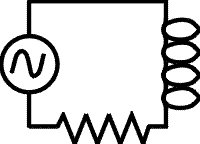Problem:

a.) What is the impedance of the circuit to the right if: f = 60 Hz, L = 20 mH, R = 4.0 W?

Solution:Z = 8.54 W

b.) If the r.m.s. voltage of the source is Vrms = 110 V, what is the r.m.s. current?

Solution: Irms = Vrms/ Z

Irms = 12.9 amps

c.) What is the peak current?

Solution: Ip = sqrt(2) ·Irms

Ip = 18.2 amps

d.) What is the power dissipated in the resistor?

Solution: P = I2rms·R

P = 1320 W

Example #4

Problem: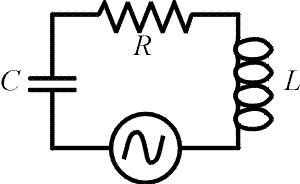a.) What is the capacitance such that the current through the circuit is a maximum?

DATA: f = 60 Hz, L = 20 mH, R = 4.0 W, Vrms = 110 V?

Solution: Choose the capacitance such that the reactance of the capacitor cancels the reactance of the inductor: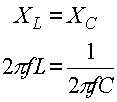C = 8.8E-5 F

b.) What is the r.m.s. current through the circuit for the capacitance found in part a?

Solution: The impedance of of the circuit is determined completely by the resistor at resonance, Z = R. The current is then V/R.

I = 27.5 Amps

c.) Find the capacitance to make the impedance equal to 8 Ohms.

Solution: The impedance is: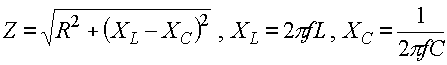First solve for the reactance of the capacitor (Xc = .612 Ohms), then find C.

C = 4.33E-3 F

Examples       AC circuits index        Lecture index